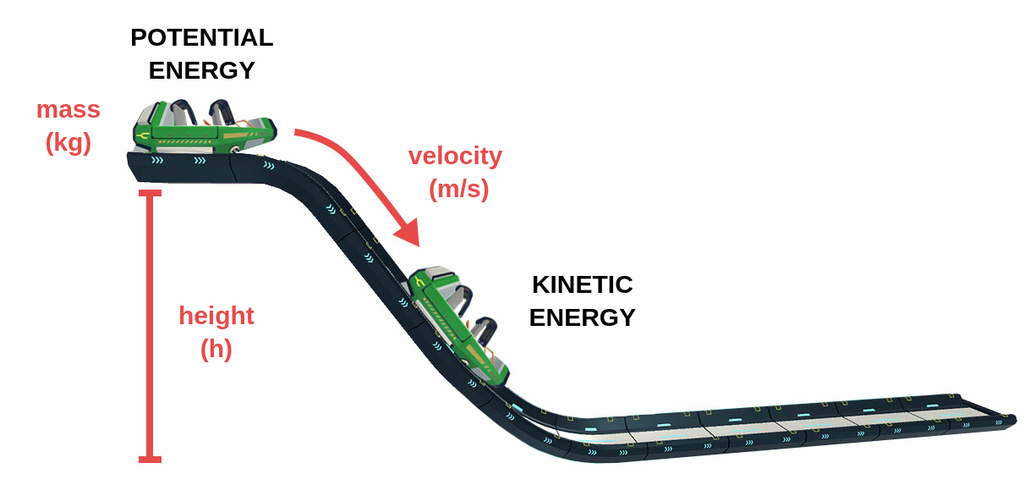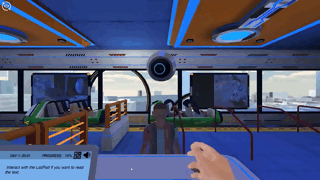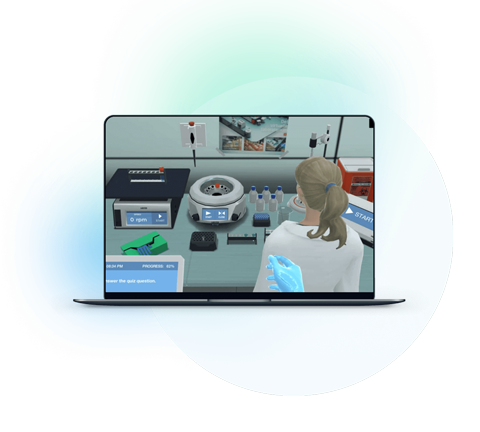Blog

# 5 Ways to Get Students Energized About Conservation of Energy

Teaching with Labster
October 18, 2022

Energy is the capability of an object to do some work. Everything possesses some form of energy; it may be potential due to position, kinetic due to its motion, or it may be chemical energy due to its bond.

The important fact about energy, as stated in the energy conservation principle, is that energy can neither be created nor destroyed. It just changes from one form to another. So, the total energy of the universe remains constant.

If you look around you, you will find numerous such devices that operate on this principle of conservation of energy. For instance, the fan cooling your room is converting electrical energy into mechanical energy. The electric lamp changes the same energy into light and heat energy.

Whenever we say we generate power, we actually mean that we are converting one form into a more useful form. For instance, in hydropower plants, we use the water’s potential energy and change it into a useful form, electrical energy, which lights up our homes.

Energy conservation is the most applicable principle in our daily lives. So, it is one of the fundamental concepts that you will have to teach in the fundamental physics course.

However, some students may find it challenging. Read on to learn reasons why students find it tricky and five techniques you can employ to make it more interesting for your students.

## Why energy conservation can be tricky for Students?

Physics, in general, is quite an interesting subject. However, at times, students find some of the topics tricky. Here’s a list of reasons why students find the conservation of energy tricky.

### 1. Energy is abstract

Energy is an abstract concept; it is the capability of an object to do work. You can mention that this object has some potential energy; however, you cannot show it to students. So, the results of energy are visible but it itself is invisible.

### 2. It’s a content-heavy topic

Energy is not a singular topic since energy exists in various forms. There are mechanical, electrical, potential, kinetic, chemical, and sound energies. Studying all these forms and their interconversion can be challenging for students.

### 3. Understanding energy requires critical thinking

We regularly use the words energy production or generation, which are technically wrong in the context of the energy conversation principle, which states that energy can neither be created nor destroyed; it just changes from one form to another. When you use the term production; students are confused about how energy is generated against the conservation principle.

## 5 Ways to make Conservation of Energy More Interesting for Students

Since we have covered the three ways why the conservation of energy can be tricky for students/ Here are five ways through which you can make energy conservation more approachable for students.

### 1. Explain in Detail the Types of Energy

In order to mention how one energy is converted into the other, students must be aware of each individual form. So, mention all the common forms of energy.

Potential Energy is the energy stored in an object due to its relative position with respect to other objects or due to its inner stresses. The common potential energy type we use is gravitational potential energy. It’s the energy due to the position relative to the earth’s surface. To calculate gravitational potential energy, we use the formula: “mgh”. Here “m” is the mass, “g” is the gravitational acceleration and “h” is the height of an object above the Earth’s surface.

Kinetic Energy is the energy possessed by a body when its in motion. It is half the product of mass and the square of velocity.

Chemical Energy is the energy stored in the form of chemical bonds. When they break, this energy is released. Fuel, food, and batteries are a source of chemical energy.

Electrical Energy is the energy due to the motion of charged particles i.e. electrons. This energy is commonly used to light up our homes. It is the one form, which can be converted to all other forms easily.

Sound energy is produced by the vibration of objects. Nuclear Energy is released during the nuclear fission and fusion reactions.

Heat energy is due to the vibrations of atoms of a substance. Most of the energy waste is in the form of heat energy.

### 2. Ask them to Locate The Energy Converters Around Them

The best way to explain any theoretical concept is by relating it to the real world. For instance, you can ask students to mention some of the energy converters around them. Initially, you can quote a few from your side and then take their response. In this way, you can encourage their participation in the discussion.

Here are a few examples of energy converters around us:

Electric Bulb

An electric bulb takes in electrical energy as input and then changes it into light and heat energy.

Electrical Energy (Main Supply Line)  = Light and Heat Energy

An Automobile

In the automobile, the chemical energy stored in the fuel is changed into the kinetic energy of the moving piston. Some part of the chemical energy is wasted as heat and sound energy, in the form of exhaust gases and engine noise.

Chemical Energy (Fuel) = Kinetic Energy (Reciprocating piston) + Heat Energy (Exhaust Gases) + Sound Energy (Engine Sound)

A Hydroelectric PowerPlant

Water stored at a height has potential energy. When it flows downhill, this energy changes to kinetic energy. The kinetic energy changes into electrical energy as it turns the huge turbine. Again, some part is wasted in the form of heat and sound energy.

Potential Energy (Water) = Kinetic Energy (Water Flow) + Electrical Energy (Turbine Rotation) + Heat Energy (Friction)

### 3. Use Analogies to Explain the Interconversion of Energy

The energy conversation principle may sound confusing since it states energy is neither created nor destroyed; it just changes its forms and transfers from system to system.

You can use different analogies to explain this principle. For example, you can use the analogy story provided by Nobel Laureate, Richard Feynman. He gave a story about wooden blocks to explain the complex process of energy conversion. His story goes like this:

A mother buys her child a set of 28 wooden blocks. These blocks are indestructible and cannot be divided. The mother keeps checking on them and counting them every day. They were always 28 in number, no matter how the child played.

One day, when she counts the blocks, they are 27. She searches the home and finds one under the rug. The count again becomes 28. The next day, she finds the blocks are 30. The son tells him his friend was there to play, so the extra blocks might be his. She returns those blocks, and the total count remains at 28.

One day, the mother searches for blocks but can only find 25 before spotting a box in the space. Although she is unable to open the box, she creates an expression for the blocks using the weights of each block and the box.

The story continues, and each day some of the blocks get hidden in different places. She keeps on adding this information to her expression. The expression becomes more complex each day. However, the block count remains constant.

The same goes with energy, it changes from one form to another and that interconversion may become complex, but the total energy remains constant.

Another analogy can be a bank account. Let’s say you have \$50,000 in your account. You purchase something and that amount is deducted from your account. In this case, you don’t say your money was destroyed. It was just transferred to the seller’s account, and you got an item in return. That’s how energy gets converted from one form to another, based on our needs.

### 4. Let them Solve Some Problems

Another way to explain physics principles is by letting them solve problems related to the conservation of energy. For instance, one problem can be:A roller coaster of mass 100 kg, at a height of 10 meters, slides down with a velocity of 14 m/s.  Calculate its total energy at the start and when it reaches the ground.

Now, before the roller coaster starts to slide down, it's completely at rest. The only energy it carries is potential energy due to the height “h”. So, here the total energy is:

T.E = K.E + P.E

T.E  =  P.E  (v=0)

T.E =  mgh =100 x 10 x 9.8 = 9800 J

When the coaster starts to slide down, its potential energy starts changing into kinetic energy. As soon as it reaches the ground, all of its potential energy has been converted into kinetic energy. Hence, the total energy is:

T.E = K.E + P.E

= K.E  (h=0)

=1/2 mv2

= ½ (100) x (14 x 14) = 9800 J

So, from this problem, it can be concluded that energy just converts from one form to another. However, the total energy of the system still remains constant.

### 5. Use Simulations to Visually Explain

One way is to design physical activities and calculate the total energy at different points along the path. However, you can use simulations to make activities more interesting and fun.

Here’s where Labster simulation can help. We have specifically designed interactive simulations for science students. These simulations through different visual games help students comprehend complex concepts. All our simulations are based on a story that is set up in a virtual world; students become a part of it and learn along their journey.

For the current case, you can check out our Conservation of Energy simulation, in which the student's mission is to raise the roller coaster's speed to 100 km/h. They do this by manipulating different forms of energy.Have any further questions regarding our simulations? Contact us now. We are here to help.What exactly are virtual labs?